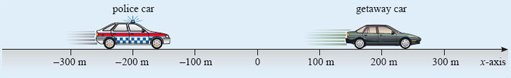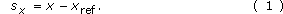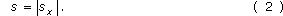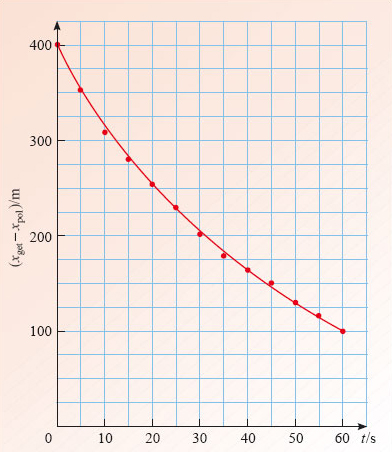Describing motion along a line

Start this free course now. Just create an account and sign in. Enrol and complete the course for a free statement of participation or digital badge if available.

Free course

# 2.4 Displacement-time graphsFigure 10: A police car in pursuit of a getaway car

A particle's position, x, is always measured from the origin of the coordinate system. However, in describing real motions it is often important to know where something is located relative to a point other than the origin. Figure 10 shows a case in point; in a high-speed pursuit neither the police nor the robbers are likely to be very interested in their location relative to the origin, but both will be interested in the location of their own vehicle relative to the other. The physical quantity used to describe the location of one point relative to another is called displacement. In the case of Figure 10, the displacement of the getaway car from the police car is 400 m and the displacement of the police car from the getaway car is −400 m. In each case the displacement of a body is determined by subtracting the position coordinate of the reference body from the position coordinate of the body of interest. Thus the displacement (measured along the x-axis) of a particle with position x from a chosen reference point with position xref is given byNotice that displacements, like positions, may be positive or negative depending on the direction of the displacement. Thus sx is always positive when x is to the right of xref and is negative when x is to the left of xref. Also note that since displacements are measured along a definite axis it makes sense to represent them by a symbol, sx, that includes a reference to that axis. This symbol may be read as 's subscript x' or, more simply, as '﻿s sub x﻿'.

A special case of Equation 1 is when the reference point xref is the origin. Then xref = 0 and sx = x. Thus the displacement of a point from the origin is the position coordinate of the point. Once we know the displacement sx of one object from another, it is easy to work out the distance s between those two objects. The distance is just the numerical value of the displacement, including the unit of measurement but ignoring any overall negative sign. So, when the displacement of the police car from the getaway car is sx = −400 m, the distance between them is s = 400 m. We describe this simple relationship between distance and displacement by saying that the distance between two objects is given by the magnitude of the displacement of one from the other, and we indicate it mathematically by writingThe two vertical bars | | constitute a modulus sign and indicate that you should work out the value of whatever they enclose and then take its magnitude (i.e. ignore any overall minus sign).

## Question 4

In Figure 8, what is the displacement sx of the car from the pedestrian, and what is the distance s between them?

sx = 50 m, and s = 50 m.

In many circumstances it is more valuable to plot a displacement-time graph rather than a position-time graph. In order to do this you either have to know the relevant displacements at various times, or you need to know enough about the positions of both the bodies involved to work out the displacements. Table 3 contains some plausible data about the positions of the two cars in Figure 10; use it to answer the following question.

t/sxpol/mxget/m
0−200200
5−115237
10−45265
1510293
2062317
25108337
30149351
35184364
40213377
45239388
50263394
55283399
60300400

## Question 5

Plot a graph to show how the displacement of the getaway car from the police car depends on time.

Since we are interested in the displacement of the getaway car from the police car, we need to plot xgetxpol. You may find it useful to tabulate these values by adding another column (headed x﻿getx﻿pol) to Table 3. The result of the plot is given in Figure 11.Figure 11: A displacement-time graph based on Table 3
S207_2